Successfully reported this slideshow.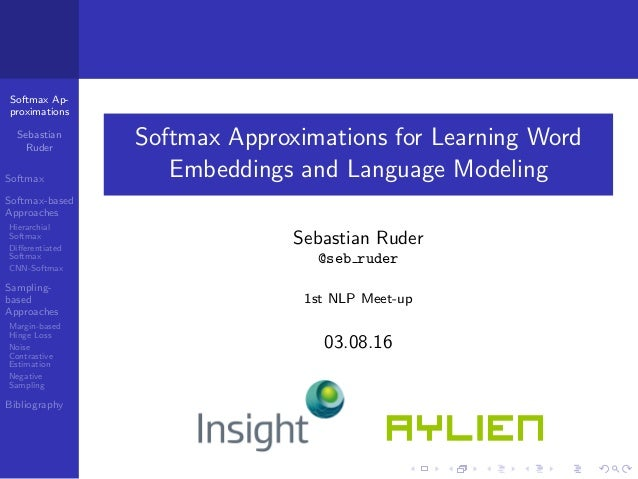Upcoming SlideShare
×

of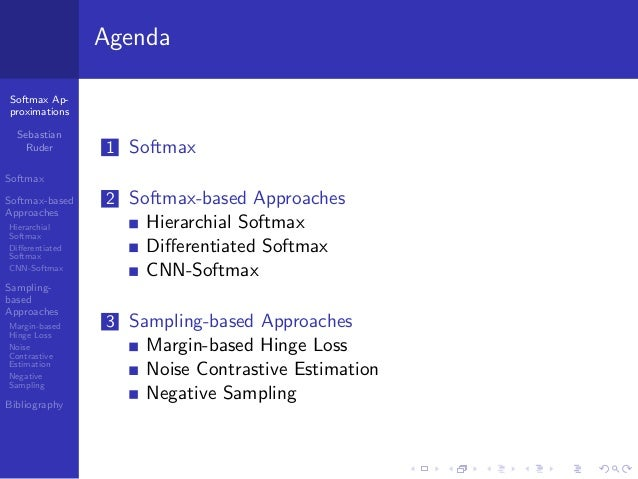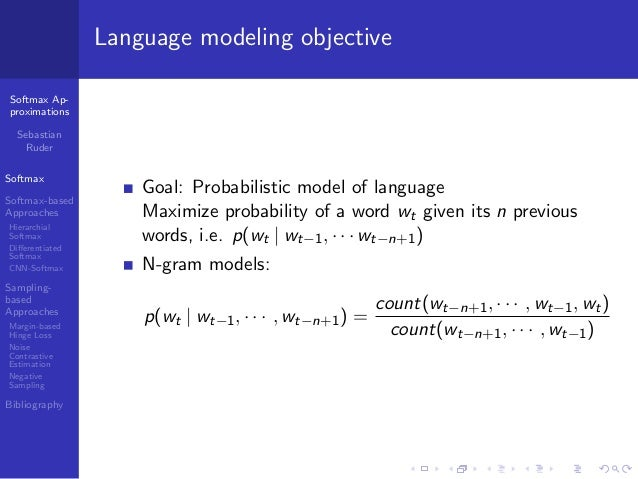3

Share

# Softmax Approximations for Learning Word Embeddings and Language Modeling (Sebastian Ruder)

Talk given at first NLP Dublin Meetup (http://www.meetup.com/NLP-Dublin/events/232278017/) by Sebastian Ruder, PhD Student at Insight Centre, Research Scientist at Aylien.

See all

See all

### Softmax Approximations for Learning Word Embeddings and Language Modeling (Sebastian Ruder)

1. 1. Softmax Ap- proximations Sebastian Ruder Softmax Softmax-based Approaches Hierarchial Softmax Diﬀerentiated Softmax CNN-Softmax Sampling- based Approaches Margin-based Hinge Loss Noise Contrastive Estimation Negative Sampling Bibliography Softmax Approximations for Learning Word Embeddings and Language Modeling Sebastian Ruder @seb ruder 1st NLP Meet-up 03.08.16
2. 2. Softmax Ap- proximations Sebastian Ruder Softmax Softmax-based Approaches Hierarchial Softmax Diﬀerentiated Softmax CNN-Softmax Sampling- based Approaches Margin-based Hinge Loss Noise Contrastive Estimation Negative Sampling Bibliography Agenda 1 Softmax 2 Softmax-based Approaches Hierarchial Softmax Diﬀerentiated Softmax CNN-Softmax 3 Sampling-based Approaches Margin-based Hinge Loss Noise Contrastive Estimation Negative Sampling
3. 3. Softmax Ap- proximations Sebastian Ruder Softmax Softmax-based Approaches Hierarchial Softmax Diﬀerentiated Softmax CNN-Softmax Sampling- based Approaches Margin-based Hinge Loss Noise Contrastive Estimation Negative Sampling Bibliography Language modeling objective Goal: Probabilistic model of language Maximize probability of a word wt given its n previous words, i.e. p(wt | wt−1, · · · wt−n+1) N-gram models: p(wt | wt−1, · · · , wt−n+1) = count(wt−n+1, · · · , wt−1, wt) count(wt−n+1, · · · , wt−1)
4. 4. Softmax Ap- proximations Sebastian Ruder Softmax Softmax-based Approaches Hierarchial Softmax Diﬀerentiated Softmax CNN-Softmax Sampling- based Approaches Margin-based Hinge Loss Noise Contrastive Estimation Negative Sampling Bibliography Softmax objective for language modeling Figure: Predicting the next word with the softmax
5. 5. Softmax Ap- proximations Sebastian Ruder Softmax Softmax-based Approaches Hierarchial Softmax Diﬀerentiated Softmax CNN-Softmax Sampling- based Approaches Margin-based Hinge Loss Noise Contrastive Estimation Negative Sampling Bibliography Softmax objective for language modeling Neural networks with softmax: p(w | wt−1, · · · , wt−n+1) = exp(h vw ) wi ∈V exp(h vwi ) where h is ”hidden” representation of input, i.e. previous words of dimensionality d vwi is the ”output” word embedding of word i, = word embedding V is the vocabulary Inner product h vw computes score (”unnormalized” probability) of model for word w given input Output word embeddings are stored in a d × |V | matrix
6. 6. Softmax Ap- proximations Sebastian Ruder Softmax Softmax-based Approaches Hierarchial Softmax Diﬀerentiated Softmax CNN-Softmax Sampling- based Approaches Margin-based Hinge Loss Noise Contrastive Estimation Negative Sampling Bibliography Neural language model Figure: Neural language model [Bengio et al., 2003]
7. 7. Softmax Ap- proximations Sebastian Ruder Softmax Softmax-based Approaches Hierarchial Softmax Diﬀerentiated Softmax CNN-Softmax Sampling- based Approaches Margin-based Hinge Loss Noise Contrastive Estimation Negative Sampling Bibliography Softmax use cases Maximum entropy models minimize same probability distribution: Ph(y | x) = exp(h · f (x, y)) y ∈Y exp(h · f (x, y )) where h is a weight vector f (x, y) is a feature vector Pervasive use in NNs: Go-to multi-class classiﬁcation objective ”Soft” selection e.g. for attention, memory retrieval, etc. Denominator is called partition function: Z = wi ∈V exp(h vwi )
8. 8. Softmax Ap- proximations Sebastian Ruder Softmax Softmax-based Approaches Hierarchial Softmax Diﬀerentiated Softmax CNN-Softmax Sampling- based Approaches Margin-based Hinge Loss Noise Contrastive Estimation Negative Sampling Bibliography Softmax-based vs. sampling-based Softmax-based approaches keep softmax layer intact, make it more eﬃcient. Sampling-based approaches optimize a diﬀerent loss function that approximates the softmax.
9. 9. Softmax Ap- proximations Sebastian Ruder Softmax Softmax-based Approaches Hierarchial Softmax Diﬀerentiated Softmax CNN-Softmax Sampling- based Approaches Margin-based Hinge Loss Noise Contrastive Estimation Negative Sampling Bibliography Hierarchical Softmax Softmax as a binary tree: evaluate at most log2 |V | nodes instead of all |V | nodes Figure: Hierarchical softmax [Morin and Bengio, 2005]
10. 10. Softmax Ap- proximations Sebastian Ruder Softmax Softmax-based Approaches Hierarchial Softmax Diﬀerentiated Softmax CNN-Softmax Sampling- based Approaches Margin-based Hinge Loss Noise Contrastive Estimation Negative Sampling Bibliography Hierarchical Softmax Structure is important; fastest (and most commonly used) variant: Huﬀman tree (short paths for frequent words) Figure: Hierarchical softmax [Mnih and Hinton, 2008]
11. 11. Softmax Ap- proximations Sebastian Ruder Softmax Softmax-based Approaches Hierarchial Softmax Diﬀerentiated Softmax CNN-Softmax Sampling- based Approaches Margin-based Hinge Loss Noise Contrastive Estimation Negative Sampling Bibliography Diﬀerentiated Softmax Idea: We have more knowledge (co-occurrences, etc.) about frequent words, less about rare words → words that occur more often allows us to ﬁt more parameters; extremely rare words only allow to ﬁt a few → diﬀerent embedding sizes to represent each output word Larger embeddings (more parameters) for frequent words, smaller embeddings for rare words
12. 12. Softmax Ap- proximations Sebastian Ruder Softmax Softmax-based Approaches Hierarchial Softmax Diﬀerentiated Softmax CNN-Softmax Sampling- based Approaches Margin-based Hinge Loss Noise Contrastive Estimation Negative Sampling Bibliography Diﬀerentiated Softmax Figure: Diﬀerentiated softmax [Chen et al., 2015]
13. 13. Softmax Ap- proximations Sebastian Ruder Softmax Softmax-based Approaches Hierarchial Softmax Diﬀerentiated Softmax CNN-Softmax Sampling- based Approaches Margin-based Hinge Loss Noise Contrastive Estimation Negative Sampling Bibliography CNN-Softmax Idea: Instead of learning all output word embeddings separately, learn function to produce them Figure: CNN-Softmax [Jozefowicz et al., 2016]
14. 14. Softmax Ap- proximations Sebastian Ruder Softmax Softmax-based Approaches Hierarchial Softmax Diﬀerentiated Softmax CNN-Softmax Sampling- based Approaches Margin-based Hinge Loss Noise Contrastive Estimation Negative Sampling Bibliography Sampling-based approaches Sampling-based approaches optimize a diﬀerent loss function that approximates the softmax.
15. 15. Softmax Ap- proximations Sebastian Ruder Softmax Softmax-based Approaches Hierarchial Softmax Diﬀerentiated Softmax CNN-Softmax Sampling- based Approaches Margin-based Hinge Loss Noise Contrastive Estimation Negative Sampling Bibliography Margin-based Hinge Loss Idea: Why do multi-class classiﬁcation at all? Only one correct word, many incorrect ones. [Collobert et al., 2011] Train model to produce higher scores for correct word windows than for incorrect ones, i.e. maximize x∈X w∈V max{0, 1 − f (x) + f (x(w) )} where x is a correct window x(w) is a ”corrupted” window (target word replaced by random word) f (x) is the score output by the model
16. 16. Softmax Ap- proximations Sebastian Ruder Softmax Softmax-based Approaches Hierarchial Softmax Diﬀerentiated Softmax CNN-Softmax Sampling- based Approaches Margin-based Hinge Loss Noise Contrastive Estimation Negative Sampling Bibliography Noise Contrastive Estimation Idea: Train model to diﬀerentiate target word from noise Figure: Noise Contrastive Estimation (NCE) [Mnih and Teh, 2012]
17. 17. Softmax Ap- proximations Sebastian Ruder Softmax Softmax-based Approaches Hierarchial Softmax Diﬀerentiated Softmax CNN-Softmax Sampling- based Approaches Margin-based Hinge Loss Noise Contrastive Estimation Negative Sampling Bibliography Noise Contrastive Estimation Language modeling reduces to binary classiﬁcation Draw k noise samples from a noise distribution (e.g. unigram) for every word; correct words given their context are true (y = 1), noise samples are false (y = 0) Minimize cross-entropy with logistic regression loss Approximates softmax as number of noise samples k increases
18. 18. Softmax Ap- proximations Sebastian Ruder Softmax Softmax-based Approaches Hierarchial Softmax Diﬀerentiated Softmax CNN-Softmax Sampling- based Approaches Margin-based Hinge Loss Noise Contrastive Estimation Negative Sampling Bibliography Negative Sampling Simpliﬁcation of NCE [Mikolov et al., 2013] No longer approximates softmax as goal is to learn high-quality word embeddings (rather than language modeling) Makes NCE more eﬃcient by making most expensive term constant
19. 19. Softmax Ap- proximations Sebastian Ruder Softmax Softmax-based Approaches Hierarchial Softmax Diﬀerentiated Softmax CNN-Softmax Sampling- based Approaches Margin-based Hinge Loss Noise Contrastive Estimation Negative Sampling Bibliography Thank you for your attention! The content of most of these slides is also available as blog posts at sebastianruder.com. For more information: sebastian@aylien.com
20. 20. Softmax Ap- proximations Sebastian Ruder Softmax Softmax-based Approaches Hierarchial Softmax Diﬀerentiated Softmax CNN-Softmax Sampling- based Approaches Margin-based Hinge Loss Noise Contrastive Estimation Negative Sampling Bibliography Bibliography I [Bengio et al., 2003] Bengio, Y., Ducharme, R., Vincent, P., and Janvin, C. (2003). A Neural Probabilistic Language Model. The Journal of Machine Learning Research, 3:1137–1155. [Chen et al., 2015] Chen, W., Grangier, D., and Auli, M. (2015). Strategies for Training Large Vocabulary Neural Language Models. [Collobert et al., 2011] Collobert, R., Weston, J., Bottou, L., Karlen, M., Kavukcuoglu, K., and Kuksa, P. (2011). Natural Language Processing (almost) from Scratch. Journal of Machine Learning Research, 12(Aug):2493–2537.
21. 21. Softmax Ap- proximations Sebastian Ruder Softmax Softmax-based Approaches Hierarchial Softmax Diﬀerentiated Softmax CNN-Softmax Sampling- based Approaches Margin-based Hinge Loss Noise Contrastive Estimation Negative Sampling Bibliography Bibliography II [Jozefowicz et al., 2016] Jozefowicz, R., Vinyals, O., Schuster, M., Shazeer, N., and Wu, Y. (2016). Exploring the Limits of Language Modeling. [Mikolov et al., 2013] Mikolov, T., Chen, K., Corrado, G., and Dean, J. (2013). Distributed Representations of Words and Phrases and their Compositionality. NIPS, pages 1–9. [Mnih and Hinton, 2008] Mnih, A. and Hinton, G. E. (2008). A Scalable Hierarchical Distributed Language Model. Advances in Neural Information Processing Systems, pages 1–8.
22. 22. Softmax Ap- proximations Sebastian Ruder Softmax Softmax-based Approaches Hierarchial Softmax Diﬀerentiated Softmax CNN-Softmax Sampling- based Approaches Margin-based Hinge Loss Noise Contrastive Estimation Negative Sampling Bibliography Bibliography III [Mnih and Teh, 2012] Mnih, A. and Teh, Y. W. (2012). A Fast and Simple Algorithm for Training Neural Probabilistic Language Models. Proceedings of the 29th International Conference on Machine Learning (ICML’12), pages 1751–1758. [Morin and Bengio, 2005] Morin, F. and Bengio, Y. (2005). Hierarchical Probabilistic Neural Network Language Model. Aistats, 5.
•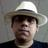#### matiskay

Oct. 13, 2017
•#### KarlLee11

Jun. 6, 2017
•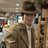Mar. 9, 2017

Total views

1,437

On Slideshare

0

From embeds

0

Number of embeds

1

42

Shares

0